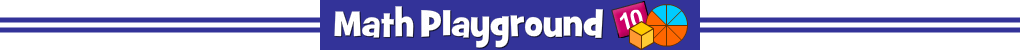AdvertisementSpeedway Fractions
NUMBER OF PLAYERS: 12
Add and subtract fractions. Simplify your answer.
Power up your racecar and win first place!
Speedway Fractions - Learning Connections
Essential Skills
Mental Math - add and subtract fractions
Problem Solving - identify equivalent fractions

Common Core Connection for Grades 3 and 4
Recognize and generate simple equivalent fractions, e.g., 1/2 = 2/4, 4/6 = 2/3.
Compare two fractions with different numerators and different denominators.
Adding and subtracting parts of the same whole.
More Math Games to Play